What does it do?

Gets the number of days between two dates

Formula breakdown:

=DAYS(end_date, start_date)

What it means:

=DAYS(ending date, starting date)

Have two dates that you want to check what is the difference in days? No problem! Excel’s DAYS Formula will compute this easily for you!

I explain how you can do this below:STEP 1: We need to enter the DAYS function in a blank cell:

## =DAYS(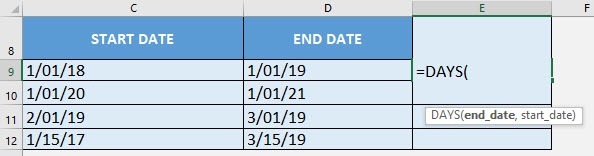STEP 2: The DAYS arguments:

## end_date

What is the ending date?

Select the cell containing the ending date:

## =DAYS(D9,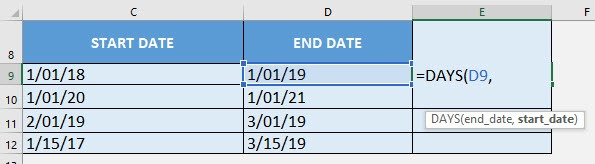## start_date

What is the starting date?

Select the cell containing the starting date:

## =DAYS(D9, C9)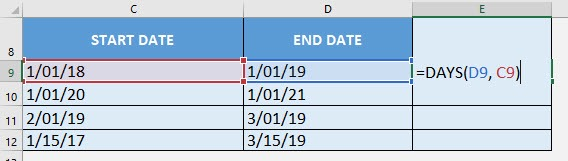Apply the same formula to the rest of the cells by dragging the lower right corner downwards.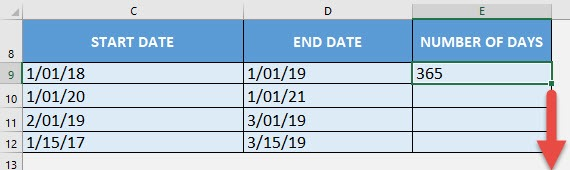You now have your differences in days!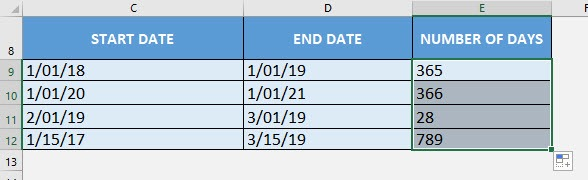How to Use the DAYS Formula in Excel